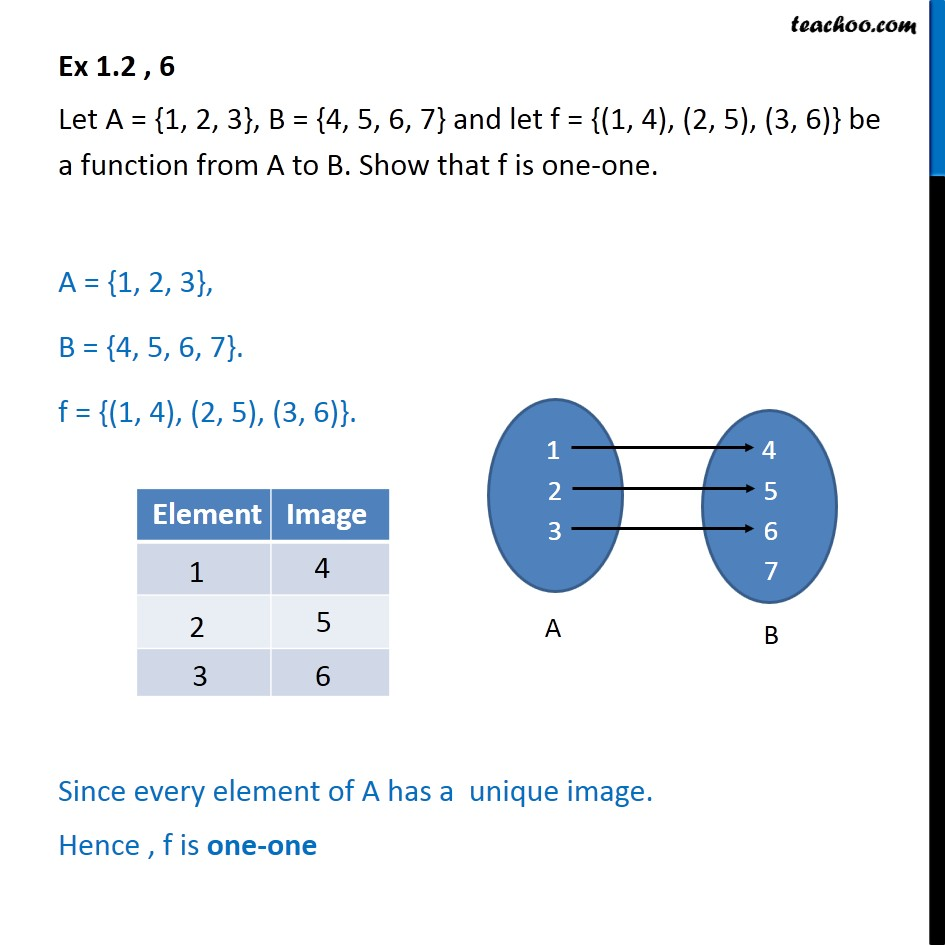1. Chapter 1 Class 12 Relation and Functions (Term 1)
2. Serial order wise
3. Ex 1.2

Transcript

Ex 1.2 , 6 Let A = {1, 2, 3}, B = {4, 5, 6, 7} and let f = {(1, 4), (2, 5), (3, 6)} be a function from A to B. Show that f is one-one. A = {1, 2, 3}, B = {4, 5, 6, 7}. f = {(1, 4), (2, 5), (3, 6)}. Since every element of A has a unique image. Hence , f is one-one